# Scale Creation¶

There are several scale-creation functions in the package. They are found in pytuning.scales and can be imported into the program’s namespace with

from pytuning.scales import *


(Note that for interactive use these are imported by default).

## The Harmonic Scale¶

We’ll start with the harmonic scale; it will illustrate many of the concepts used in scale creation.

There are two important concepts to understand:

• Normalization: If a scale is normalized (which is the default in all cases), then the intervals of the scale are normalized to fall within a single octave. This means scaling the interval either up or down the number of octaves needed to make the interval fall between the unison and the octave.
• The Octave: Normally an octave is defined as a doubling of frequency (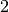), but it is possible to define an octave by some other number. If this is the case the normalization will takes place over this new octave.

The function to create a harmonic scale is, create_harmonic_scale:

As an example, if we create a non-normalized harmonic scale of 10 harmonics:

harmonic_scale = create_harmonic_scale(1, 10, normalize=False)


We have the following scale: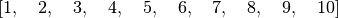If we normalize it each interval is scaled by a power of two to fall within 1 and 2. So, for example, the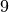becomes, because the nine must be scaled by three octaves to fall within that range: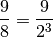So the normalized scale is: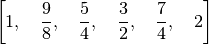But if we change our octave definition to be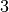, we normalize on powers of 3:

harmonic_scale = create_harmonic_scale(1, 10, octave=3)


yields: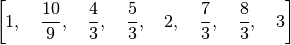## Equal Divsion of the Octave (Equal Temprament)¶

Equal temperament scales can be created with the create_edo_scale() function. Note that this function does not accept a normalize argument, because EDO scales are normalized by definition. If does, however, allow you to change the definition of the formal octave.

## Scales from a Generator Interval¶

The create_equal_interval_scale() function will generate a scale from a generator interval. This is the base function for several other scale types (for example, the Pythagorean scale is created with a generator interval of).

In he creation of a scale, the generator interval can either be used directly (for, for example, making each successive tone a generator interval above the previous tone), or in an inverted sense (making each interval a generator down from the previous). This function starts from the unison and walks down the number specified, walking up for the rest of the intervals.

## The Pythagorean Scale¶

This is the standard Pythagorean scale. Note that we can choose the number of up and down intervals in the scale. The default yields the standard scale, with the fourth degree as a diminished fifth, as opposed to the augmented fourth.

So, for the standard scale we can use:

scale = create_pythagorean_scale()


yielding: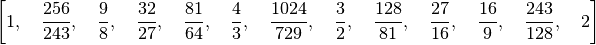If we wanted the augmented fourth:

scale = create_pythagorean_scale(number_down_fifths=5)


yielding: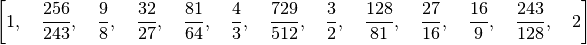## The Quarter-Comma Meantone Scale¶

An example of use:

scale = create_quarter_comma_meantone_scale()


yields: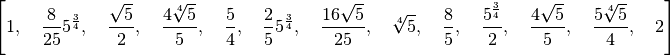## Diatonic Scales¶

As another example of creating a diatonic scale, we can use the five-limit constructors (which are defined in pytuning.constants):

five_limit_constructors = [
(sp.Rational(16,15), "s"),
(sp.Rational(10,9),  "t"),
(sp.Rational(9,8),   "T"),
]


to create Ptolemy’s Intense Diatonic Scale:

from pytuning.constants import five_limit_constructors
from pytuning.scales import create_diatonic_scale

scale = create_diatonic_scale(five_limit_constructors,
["T", "t", "s", "T", "t", "T", "s"])


which gives us: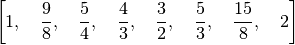Note that if every identifier is a single-character string, specification can also be passed in as a string. So this is equivalent:

from pytuning.constants import five_limit_constructors
from pytuning.scales import create_diatonic_scale

scale = create_diatonic_scale(five_limit_constructors, "TtsTtTs")